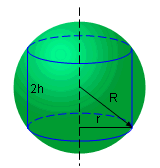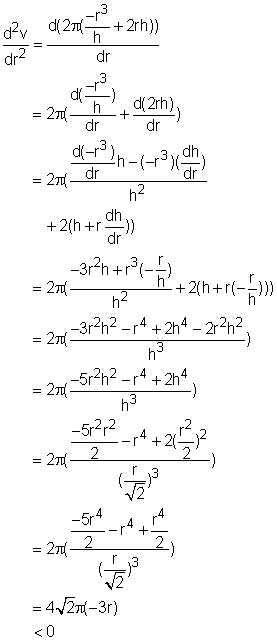Ch 4. The Mean Value Theorem Multimedia Engineering Math Maximum & Minimum Rolle'sTheorem Mean ValueTheorem MonotonicFunctions First DerivativeTest Concavity &Inflection SecondDerivative Test
 Chapter 1. Limits 2. Derivatives I 3. Derivatives II 4. Mean Value 5. Curve Sketching 6. Integrals 7. Inverse Functions 8. Integration Tech. 9. Integrate App. 10. Parametric Eqs. 11. Polar Coord. 12. Series Appendix Basic Math Units Search eBooks Dynamics Fluids Math Mechanics Statics Thermodynamics Author(s): Hengzhong Wen Chean Chin Ngo Meirong Huang Kurt Gramoll ©Kurt GramollMATHEMATICS - CASE STUDY SOLUTIONCylinder in Sphere Find the maximum weight of a cylinder that can be embedded in a ball with 0.05 m radius. The mass density of the ball is 7,900 kg/m3. Let the radius of the ball and the cylinder be R and r respectively. Let the height of the cylinder be 2h. Let the weight, volume and mass density of the cylinder be w, v and ρ respectively. It is know that the weight of the cylinder, w, is determined by its mass density, ρ, and volume, v. That is      w = v ρ Since the mass density for a steel ball is a constant, the volume of the cylinder must be the biggest to make the its weight heaviest. Therefore, this problem turned out to be finding the the maximum volume in a ball. In geometry, the volume of the cylinder is      v = 2 π r2 h                                                (1) The Second Derivative Test states: if the first derivative of a function at a point is 0 and the second derivative at that point is less than 0, then the function has a local maximum at that point. In order to find the maximum volume in this problem, the radius where the first derivative equals 0 needs to be calculated. After that, the second derivative value with this radius needs to be evaluated and compares with 0 to determine its sign. Thus calculate the first derivative of the volume with respect to radius. That is      dv/dr = d(2π r2h) dr              =2π (r2 dh/dr + 2rh)                           (2)      As mentioned previously dv/dx needs to be 0, so      2π (r2dh/dr + 2rh) = 0                               (3) In order to solve equation (3), dh/dr must be calculated first. In geometry, the relationship between the height of the cylinder and the radius of the ball and the cylinder can be expressed as      r2 + h2 = R2                                           (4) Derivative both side of the equation (4) with respect to r gives      2r + 2h dh/dr = 0 Rearranging the equation gives      dh/dr = -r/h                                            (5) Substituting equation (5) into equation (3) to find the relationship between r and h. That is      2π (r2(-r/h) + 2rh) = 0   Rearranging the equation gives      h2 = r2/2                                                (6) Substituting equation (6) into equation (4) gives the height and the radius for the cylinder      h = (1/3)0.5 R                                          (7)      r = (2/3)0.5 R                                           (8) Therefore, (2/3)0.5R is the radius that cause the value of the first derivative to be 0. In other word, the volume with this radius have an extreme value. It might be the maximum or the minimum value. The second derivative test is capable to verify the minimum or maximum value of the volume function. The second derivative test requires to calculate the value of the second derivative of the function and compare with 0. In order to calculate the second derivative of the volume, the first derivative of the function need to be rearranged. Substituting equation (5) into equation (2) gives:      dv/dr = 2π (r2(-r/h) + 2rh)              =2π (-r3/h) + 2rh)                            (9) Derivative both side of equation (9) gives the second derivative of volume with respect to radius. That isIt has been determined that when the value of the first derivative of volume is 0, the value of the second derivative is less than 0. According to the second derivative test, the volume when r = (2/3)0.5R is maximum. Therefore, the maximum weight of this cylinder is      w = v ρ         = 2π r2 h ρ         = 2π (2/3)0.5 R2 (1/3)0.5 Rρ         = 2π (2/9)0.5 R3ρ         = 2π (2/9)0.5 (0.05)3 (7,900)         = 2.93 kg So the maximum weight of a cylinder that can be embedded in a ball with 0.05 m radius is 2.93 kg.